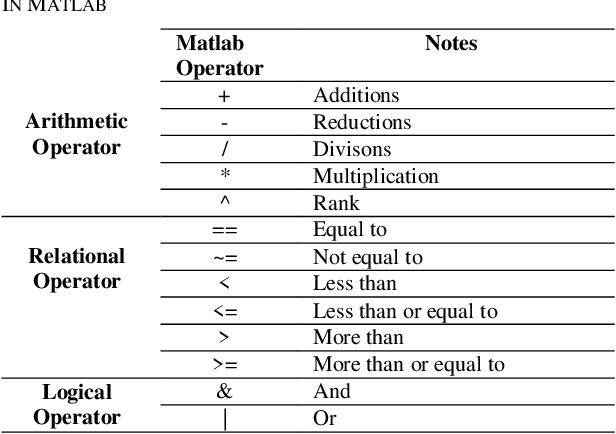Home / Technology / AI & IT / Matlab and Simulink

Modeling is the process of representing a model (e.g., physical, mathematical, or logical representation of a system, entity, phenomenon, or process) which includes its construction and working. This model is similar to a real system, which helps the analyst predict the effect of changes to the system. Simulation of a system is the operation of a model in terms of time or space, which helps analyze the performance of an existing or a proposed system. Modeling and simulation (M&S) is the use of models  as a basis for simulations to develop data utilized for managerial or technical decision making.

To transform development of complex systems, market-leading companies adopt Model-Based Design by systematically using models throughout the entire process.

• Use a virtual model to simulate and test your system early and often
• Validate your design with physical models, Hardware-in-the-Loop testing, and rapid prototyping
• Generate production-quality C, C++ , CUDA, PLC, Verilog, and VHDL code and deploy directly to your embedded system
• Maintain a digital thread with traceability through requirements, system architecture, component design, code and tests
• Extend models to systems in operation to perform predictive maintenance and fault analysis

MATLAB, which stands for MATrix LABoratory, is a technical computing environment for high-performance numeric computation and visualization. MATLAB is a Programming language and interactive environment well suited for computing, algorithms, data processing, and visualization.

SIMULINK is a part of MATLAB that can be used to simulate dynamic systems. Simulink is an Environment for graphical, model-based simulation of dynamic systems.  To facilitate the model definition, SIMULINK adds a new class of windows called block diagram windows. In these windows, models are created and edited primarily by mouse driven commands. Part of mastering SIMULINK is to become familiar with manipulating model components within these windows.

MATLAB is an abbreviation for “matrix laboratory.” While other programming languages mostly work with numbers one at a time, MATLAB® is designed to operate primarily on whole matrices and arrays. All MATLAB variables are multidimensional arrays, no matter what type of data. A matrix is a two-dimensional array often used for linear algebra. MATLAB allows you to process all of the values in a matrix using a single arithmetic operator or function."Matlab and Simulink." International Defense Security & Technology - January 31, 2023, https://idstch.com/technology/ict/matlab-and-simulink/
International Defense Security & Technology December 6, 2022 Matlab and Simulink., viewed January 31, 2023,<https://idstch.com/technology/ict/matlab-and-simulink/>
International Defense Security & Technology - Matlab and Simulink. [Internet]. [Accessed January 31, 2023]. Available from: https://idstch.com/technology/ict/matlab-and-simulink/
"Matlab and Simulink." International Defense Security & Technology - Accessed January 31, 2023. https://idstch.com/technology/ict/matlab-and-simulink/
"Matlab and Simulink." International Defense Security & Technology [Online]. Available: https://idstch.com/technology/ict/matlab-and-simulink/. [Accessed: January 31, 2023]